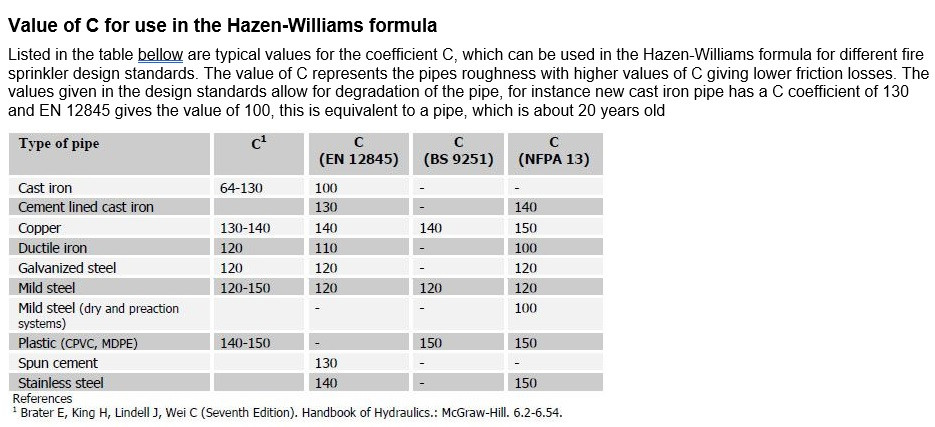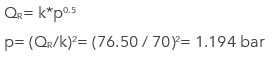Search
• Mihai

# Pressure Drop In Sprinkler Systems

Updated: Aug 4

## IntroductionOur company has an extensive expertise in concept design and design development for fire fighting systems. We decided to share some of that knowledge by publishing technical articles about topics that we feel are important.

In this write-up we will indicate how to carry on calculation/sizing for a sprinkler system. The example will consist of a three sprinkler head system branch which is part of a larger “tree” sprinkler system. A tree system is a 'end feed' system, with water being fed from one direction as opposed to a grid or loop system when water may arrive at the sprinkler head from more than one direction. The same calculation can be used for any other application where a k-factor is used.

For the calculation to take place the pipe internal diameter, length and C factor are required as per below example:Note: More about types of pipes in our article “Sprinkler piping material”.

C-factor values as per the below table:For pipe types and inner diameter see attached link.

Other important information related to the type of the sprinkler heads is included in the below table:Note: We have considered the sprinkler heads are identical for the ease of calculation. This is not always the case.

## Pressure Drop Calculation Methodology

The next step is to calculate the minimum flow rate which will be required at the most remote located sprinkler in the index run. The minimum flow rate will have to satisfy the design density of 7.5mm/min.

The required flow rate is calculated by multiplying the design density with the area the head is covering.The second stage is to calculate the flow rate for the sprinkler considering the above K - factor and head pressure.Where

p = the required pressure k = the discharge coefficient of the sprinkler (k-factor)

From the above it results that the minimum required flow rate is bigger than what the sprinkler head can provide. Te solution for this is to consider the sprinkler flow rate equal with the minimum required flow rate, keep the same k factor and find out the required pressure from the equation.We have now determined the minimum pressure and flow for the first sprinkler at the first node which will be 76.50 L/min @ 1.2 bar. The next step is to calculate the pressure drop in the pipe between node 1 and 2 and for this we will use the Hazen-Williams pressure loss formula.Where

p = pressure loss in bar per meter Q = flow through the pipe in L/min C = friction loss coefficient d = internal diameter of the pipe in mm

The pressure loss in the first pipe is 0.0242 Bar/m and the total pressure loss in the pipe is 0.0845bar.

The pressure at node 2 is equal with 1.2bar+0.0845bar=1.28bar.

The next step is to find the flow rate for the seconded sprinkler head at node 2. To do this we will use the K-Factor formula mentioned before in the above calculations.Considering the above the new flow rate in the pipe connecting node 2 with node 3 is 79.33+76.5l/min=155.835l/min

The pressure loss in the second pipe (node 2-3) is equal to 0.315bar

The pressure at node 3 is equal with 1.28bar+0.315bar=1.597bar.

We now need to find the flow rate for the sprinkler at node 3. We do this by using the same k - factor formula and the 1.597 bar pressure. This gives 70 x 1.5970.5 = 88.50 L/min from the sprinkler head at node 3.

The total flow rate resulted for the 3 sprinkler heads is 155.835+88.50l/min=244.33l/min.

The last step is to find the pressure loss in the third pipe connecting the node 3 with the main branch by again using the Hazen-Williams pressure loss formula. However, the last pipe has an internal diameter of 36.66 mm so this gives us a pressure loss of 0.19bar.

The total pressure at the branch level is 1.597+0.19bar=1.787bar

The most remote sprinkler head will match the min flow requirement whereas the rest of the sprinkler heads will have a higher pressure, discharging more water.